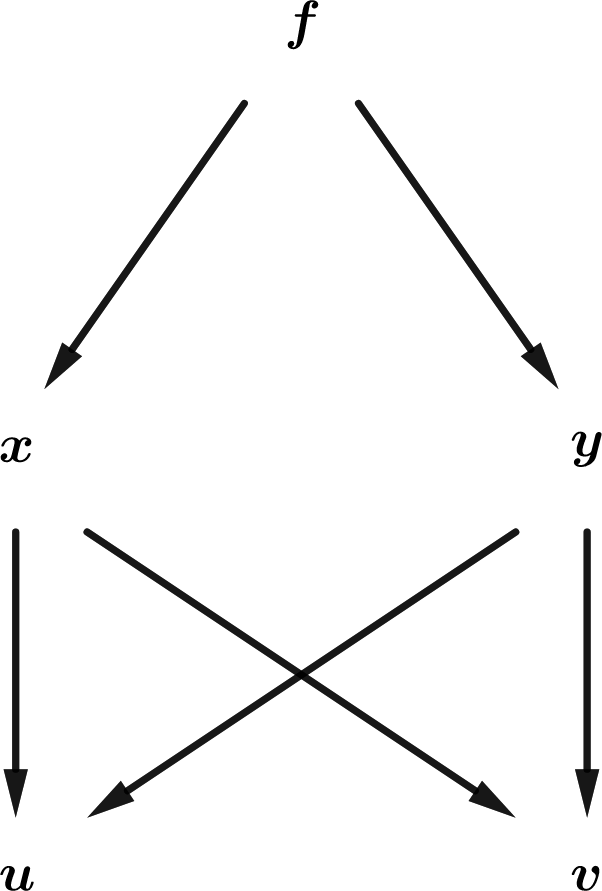## Section6.11Chain Rule via Tree DiagramsFigure 6.11.1. Tree diagrams relating $$x\text{,}$$ $$y$$ with $$u\text{,}$$ $$v\text{.}$$

Suppose $$f=f(x,y)\text{.}$$ Then of course

\begin{gather*} df = \left(\Partial{f}{x}\right)_y dx + \left(\Partial{f}{y}\right)_x dy \end{gather*}

where the subscripts keep track of which variables are being held constant when taking partial derivatives. If $$x=x(u,v)\text{,}$$ $$y=y(u,v)\text{,}$$ then

\begin{gather*} dx = \left(\Partial{x}{u}\right)_v du + \left(\Partial{x}{v}\right)_u dv \end{gather*}

with a similar expression holding for $$dy\text{.}$$ Combining these expressions and rearranging terms, we obtain

\begin{align*} df = \amp \left( \left(\Partial{f}{x}\right)_y \left(\Partial{x}{u}\right)_v + \left(\Partial{f}{y}\right)_x \left(\Partial{y}{u}\right)_v \right) du\\ \amp + \left( \left(\Partial{f}{x}\right)_y \left(\Partial{x}{v}\right)_u + \left(\Partial{f}{y}\right)_x \left(\Partial{y}{v}\right)_u \right) dv . \end{align*}

But we also know that

\begin{gather*} df = \left(\Partial{f}{u}\right)_v du + \left(\Partial{f}{v}\right)_u dv . \end{gather*}

Setting $$v=\hbox{constant}\text{,}$$ we obtain

\begin{equation} \left(\Partial{f}{u}\right)_v = \left(\Partial{f}{x}\right)_y \left(\Partial{x}{u}\right)_v + \left(\Partial{f}{y}\right)_x \left(\Partial{y}{u}\right)_v\tag{6.11.1} \end{equation}

with a similar expression holding for the derivative of $$f$$ with respect to $$v\text{.}$$

An easy way to remember such formulas is to use a tree diagram, as shown in the first diagram in Figure 6.11.1. To use a tree diagram, determine which derivative you want to take, in this case the derivative of $$f$$ with respect to $$u\text{.}$$ Now follow all possible paths from $$f$$ to $$u\text{,}$$ with each arrow corresponding to a derivative of the “top” quantity with respect to the “bottom” quantity, and where in each case the variable(s) not pointed to by the arrow are to be held constant.

However, one often wants to know how to express the derivatives of $$f$$ with respect to $$x$$ and $$y$$ in terms of its derivatives with respect to $$u$$ and $$v\text{,}$$ rather than the other way around. The argument in this case is the same, with the roles of ($$x\text{,}$$$$y$$) and ($$u\text{,}$$$$v$$) reversed, as in the tree diagram in the second diagram in Figure 6.11.1. This construction results in

\begin{equation} \left(\Partial{f}{x}\right)_y = \left(\Partial{f}{u}\right)_v \left(\Partial{u}{x}\right)_y + \left(\Partial{f}{v}\right)_u \left(\Partial{v}{x}\right)_y\tag{6.11.2} \end{equation}

with a similar expression for the derivative of $$f$$ with respect to $$y\text{.}$$ When comparing (6.11.2) with (6.11.1), it is important to realize that $$\left(\Partial{x}{u}\right)_v$$ and $$\left(\Partial{u}{x}\right)_y$$ are not necessarily reciprocals of each other.  1

Finally, it is possible to reinterpret (6.11.2) as a statement about derivative operators, rather than derivatives, simply by removing $$f\text{.}$$ Thus,

\begin{equation} \left(\Partial{}{x}\right)_y = \left(\Partial{u}{x}\right)_y \left(\Partial{}{u}\right)_v + \left(\Partial{v}{x}\right)_y \left(\Partial{}{v}\right)_u\tag{6.11.3} \end{equation}

where we have reordered the terms slightly. When using such expressions, you will often need to express the derivatives on the RHS in terms of $$u$$ and $$v$$ alone, rather than in terms of $$x$$ and $$y\text{.}$$ Having done so, it is possible to use these expressions to determine higher-order derivative operators as well, such as the Laplacian.

It turns out that the partial derivatives relating $$(x,y)$$ to $$(u,v)$$ and vice versa can be viewed as the components of a matrix, and that the two matrices are inverses of each other.